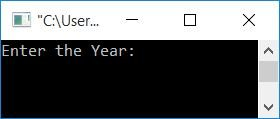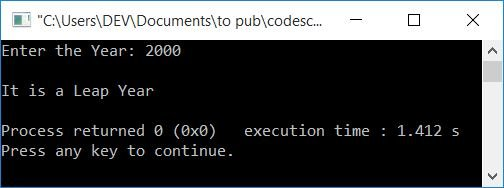# C++ program to check if a given year is a leap year or not

In this article, you will learn and get code for checking whether the given year by the user at run-time is a leap year or not in C++ programming. The leap year program is created using the following three approaches:

Before going through the program, if you are not aware of the logic used behind leap year, then I must recommend that you visit Leap Year Logic once. There, you will learn everything about the leap year. But in short, here are the rules to check for the leap year:

• It is a leap year if the year is divisible by 4, but not by 100.
• It is a leap year if the year is divisible by 400.
• And in all other cases, it is not a leap year.

## In C++, check the leap year

To check whether the input year is a leap year or not in C++ programming, you have to ask the user to enter the year first and then apply the logic and print the message as shown in the program given below:

So the question is: write a C++ program to determine whether a year is a leap year or not. The answer to this question is given below:

```#include<iostream>
using namespace std;
int main()
{
int yr;
cout<<"Enter the Year: ";
cin>>yr;
if((yr%4==0) && (yr%100!=0))
cout<<"\nIt is a Leap Year";
else if(yr%400==0)
cout<<"\nIt is a Leap Year";
else
cout<<"\nIt is not a Leap Year";
cout<<endl;
return 0;
}```

This program was built and runs under the Code::Blocks IDE. Here is its sample run:Now supply the input, that is, enter any year to print, whether it is a leap year or not, as shown in the output given below with user input as 1900:Here is another sample run with user input as 2000:Note: To be a leap year, if a year is divisible by 4, then it must not be divisible by 100.

## Leap Year Program Using Functions in C++

This is the end of this article. This program uses the user-defined function checkLeap() to check whether a given year is a leap year or not.

```#include<iostream>
using namespace std;
int checkLeap(int);
int main()
{
int yr, val;
cout<<"Enter the Year: ";
cin>>yr;
val = checkLeap(yr);
if(val==1)
cout<<"\nIt is a Leap Year";
else
cout<<"\nIt is not a Leap Year";
cout<<endl;
return 0;
}
int checkLeap(int yr)
{
if((yr%4==0) && (yr%100!=0))
return 1;
else if(yr%400==0)
return 1;
else
return 0;
}```

You can also accomplish the same goal in another way, as shown below. Rather than returning the value according to the condition, the function in this program just prints the message directly from inside the function without returning any value.

```#include<iostream>
using namespace std;
void checkLeap(int);
int main()
{
int yr, val;
cout<<"Enter the Year: ";
cin>>yr;
checkLeap(yr);
cout<<endl;
return 0;
}
void checkLeap(int yr)
{
if((yr%4==0) && (yr%100!=0))
cout<<"\nIt is a Leap Year";
else if(yr%400==0)
cout<<"\nIt is a Leap Year";
else
cout<<"\nIt is not a Leap Year";
}```

## In C++, check the leap year using Class

Now let's create the same-purpose program using classes and objects.

```#include<iostream>
using namespace std;
class CodesCracker
{
private:
int yr;
public:
int getData();
void checkLeapYear(int);
};
int CodesCracker::getData()
{
cout<<"Enter the Year: ";
cin>>yr;
return yr;
}
void CodesCracker::checkLeapYear(int yr)
{
if((yr%4==0) && (yr%100!=0))
cout<<"\nIt is a Leap Year";
else if(yr%400==0)
cout<<"\nIt is a Leap Year";
else
cout<<"\nIt is not a Leap Year";
}
int main()
{
CodesCracker c;
int y;
y = c.getData();
c.checkLeapYear(y);
cout<<endl;
return 0;
}```

This will produce the same output as the first program. Here is a very brief explanation of the above program:

• Inside the main() function, an object c of class CodesCracker is created.
• Using this object, we have called the function getData() of that class and initialized its return value to y.
• That is, inside the getData() function, the value of year gets received (by the user at run-time) and put into yr. And the yr value is returned. Therefore, now the value of yr gets initialized to y inside the main() function.
• Now inside the main() function, we have called the checkLeapYear() function of the same class using its object c.
• We've passed y as its argument. Therefore, in the checkLeapYear() function, with the given value of year (by the user), we've checked and printed the message, whether it is a leap year or not.

#### The same program in different languages

C++ Quiz

« Previous Program Next Program »